# Glossary of Physics Terms Used in the HSC

This complete glossary of Physics terms lists all the terminology you need to ace HSC Physics.

## What is glossary of Physics terms?

This complete glossary of Physics terms provides a quick reference to the definitions of commonly used physics terms in HSC Physics course.

Go to Physics term:

### AC induction motor

A three-phase AC induction motor is an AC electric motor in which the electric current in the rotor needed to produce torque is induced by electromagnetic induction from the apparent rotating magnetic field of the stator coils.

An induction motor consists of :

1. A stator consisting of multiple coils connected to a three phase power supply
2. A rotor consisting of cylinder made of metal An Axle No slip rings and brushes

An important feature of the induction motor is that power is supplied to the stator only. There is no power supply to the rotor. (Since there are no contacts allowing a current flow into the rotor coil). Instead, the current is induced in the rotor by the magnetic field of the stator.

### Activity of a radioactive sample

The activity of a radioactive sample is a measure of how “radioactive” a sample is. The activity measures the number of decays per second that occur in a sample of a radioactive material.

### Alpha particles

An alpha particle is a helium ion. Each alpha particle has a positive charge twice that of a proton and a mass four times that of a proton as it contains two protons and two neutrons. An alpha particle is not a fundamental particle.

### Ampere

The new definition of the ampere is the flow of 6.2415093 x 1018 elementary charges per second. The new definition for the ampere was approved in November 2018 and is effective from 20 May 2019.

### Ampere’s force law

Ampere’s force law or Ampere’s law describes the relationship between the force between two parallel conductors $F$ and the currents flowing through the conductors $I_{1}$ and $I_{2}$ , their separation distance $d$ and the common parallel length of the wires $l$.

Mathematically Ampere’s law is expressed as:

$\Large \frac{F}{l}=\frac{\mu_0}{2\pi} \frac{I_1I_2}{d}$

where

• $F$ = Force between the parallel conductors
• $l$ = Common length of the parallel conductors
• $I_1$ = Current flowing through one of the parallel conductors
• $I_2$ = Current flowing through the other parallel conductors
• $d$ = Distance of separation between the parallel conductors
• $\mu_0$ = Magnetic permeability constant, $4\pi \times 10^{-7} NA^{-2}$

### Angular displacement ($\theta$)

Angular displacement is the position of the object around the circle, measured as an angle. The unit of angular displacement is in radians.

### Angular velocity ($\omega$)

The rate of change of angular displacement. It measures how fast or slow an object undergoes a change in angular displacement. It is measured in radians per second.

Mathematically it is expressed as:

$\Large \omega=\frac{\Delta \theta}{\Delta t}$

### Atmospheric drag

Atmospheric drag refers to the friction due to atmosphere. All satellites in low Earth orbit are subjected to some degree of atmospheric drag.
The amount of atmospheric drag acting on a satellite depends on:

1. Density of air [which depends on altitude]
2. Speed of the satellite

### Atomic mass unit

Atomic mass unit or the unified mass unit u is an alternative unit for expressing atomic masses and is defined such that the mass of a ${12 \atop 6} C$ atom is exactly 12 u.

### Atomic spectra

Atomic spectra refer to the spectrum of light absorbed or emitted by specific elements.

There are two types of atomic spectra

1. Absorption spectra involve passing light with a wide wavelength range through a sample, and recording the spectrum of light that is transmitted.
2. Emission spectra are the spectra of light emitted by a sample after the sample has been given energy in some form.

An example of hydrogen absorption and emission spectrum is shown below.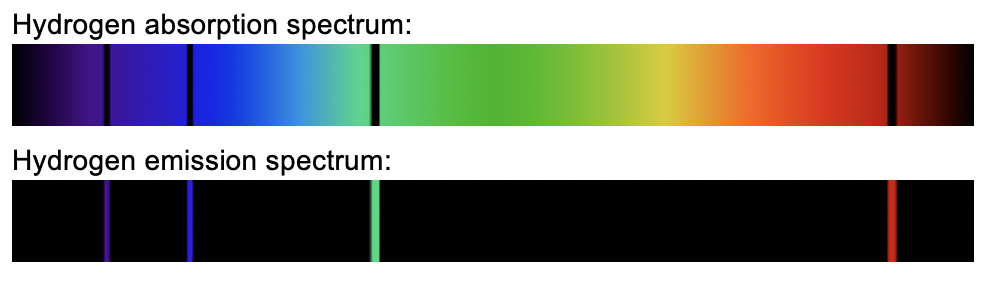### Back EMF

An induced EMF produced in an electric motor as a result of a change in flux is called a back EMF as it opposes the supply EMF (i.e. the voltage supplied by the battery.

A relationship between the back EMF and the current in the coil in a DC motor are expressed in a mathematical formula below:

$EMF_{coil}=EMF_{supply} -EMF_{back} =I_{coil}R \propto Torque$

###### To learn more about ‘Back EMF’, view Module 6 / Chapter 6 / Lesson 5 on Learnable.

A banked road is a surface that is inclined and not flat. An example of banked surfaces is Velodrome which is an arena for track cycling.

### Black body

A black body is an ideal physical object which absorbs all electromagnetic radiation incident upon it (i.e. from an external source). The term “black body” was introduced by Gustav Kirchhoff in 1862.

• A blackbody is a perfect absorber of electromagnetic radiation over all wavelengths.
• If all the incident radiation is absorbed, any electromagnetic radiation from the object must be its own (due to its temperature).
• Hence the blackbody is also a perfect emitter of electromagnetic radiation as it is capable of emitting radiation over all wavelengths.

The electromagnetic radiation emitted by a black body at a constant temperature is called black body radiation.

The graph of the distribution of intensity of radiation versus wavelength for a blackbody at four different temperatures is shown in the figure below.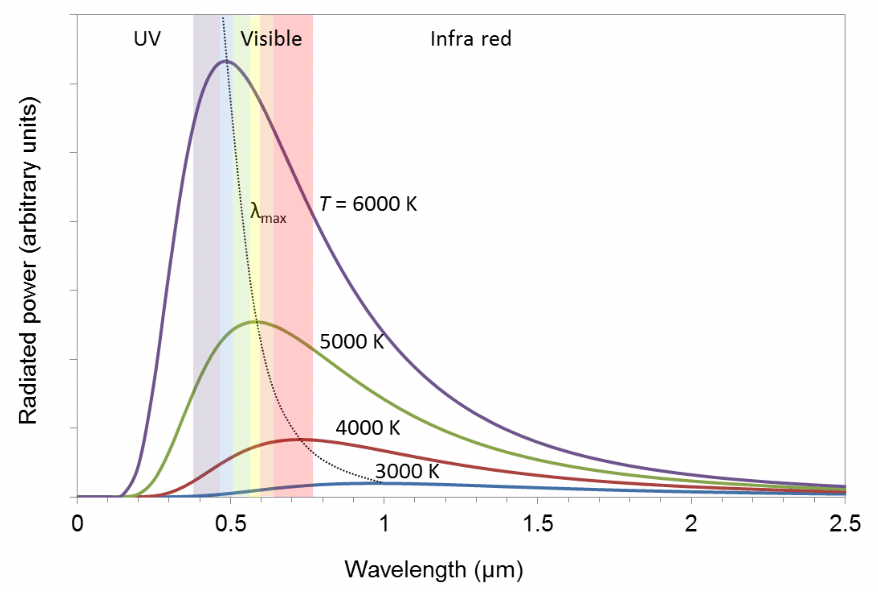### Cathode rays

Cathode rays are streams of electrons flowing from the cathode (negative electrode) towards the anode (positive electrode) in a discharge tube.

### Centripetal acceleration

Centripetal acceleration is the acceleration acting towards the centre of rotation on an object moving in a circle.

The magnitude of centripetal acceleration is given by:

$\Large a_c=\frac{v^2}{r}$

### Centripetal force

Centripetal force is the force acting on an object towards the centre of rotation required in order for the object to move in a circle.

$\Large F_c=\frac{mv^2}{r} =mr \omega ^2$

### Circular motion

Circular motion is a movement of an object along the circumference of a circle or rotation along a circular path.
It can be:

1. uniform, with constant angular rate of rotation and constant speed, or
2. non-uniform with a changing rate of rotation

### Conical pendulum

A conical pendulum consists of a mass at the end of a light string, moving at a constant speed in a circle with the string tracing out a cone as shown below.### Centrifuge

A centrifuge is an equipment that rotates an object in a circle around a fixed point in order to simulate gravity or acceleration.

### DC electric motor

A brushed DC electric motor is a simplest type of DC motor that is designed to operate on a DC power supply and consists of:

1. A rotor coils: There may be only one, in a very simple motor, or several coils, usually of several turns of insulated wire, wound onto the armature. The ends of the coils are connected to bars on the commutator.
2. Armature: Consists of a cylinder of laminated iron mounted on an axle. Often there are longitudinal grooves into which the coils are wound.
3. An axle: A cylindrical bar of hardened steel passing through the centre of the armature and the commutator.
4. A commutator: A broad ring of metal mounted on the axle at one end of the armature, and cut into an even number of separate bars (two in a simple motor). Each opposite pair of bars is connected to one coil. The commutator reverses the direction of current flow in each coil every half-revolution. This ensures that the torque on each coil is always in the same direction.
5. Brushes: Compressed carbon blocks, connected to the external circuit, mounted on opposite sides of the commutator and spring-loaded to make close contact with the commutator bars.
6. A pair of permanent magnets: Two permanent magnets on opposite sides of the motor, with opposite poles facing each other. The pole faces are curved to fit around the armature. The magnets supply the magnetic field which interacts with the current in the rotor coil(s) to produce the motor effect.
or
7. Pairs of electromagnetic coils: Each stator coil (or “field” coil) is wound on a soft iron core attached to the casing of the motor. The coils are shaped to fit around the armature.

### de Broglie’s hypothesis

Louis Victor de Broglie in 1923 postulated that because photons have wave and particle characteristics, all forms of matter have wave as well as particle properties.

According to de Broglie’s hypothesis:

1. Electrons have a dual particle/wave nature.
2. The wavelength of a particle is related to momentum by:

$​\LARGE λ_{particle}=\frac{h}{p}=\frac{h}{mv}​ ​$

where ​

• $m$ = mass of a particle,
• $​v$ = velocity of a particle ​
• $h$ = Planck’s constant and
• $​\lambda$ = wavelength.

### Diffraction of light

Diffraction is the bending of waves around an obstacle. Diffraction is only obvious when the wavelength(λ) is comparable to the size of the slit (a) or object that causes the diffraction.

When the size of the opening is similar to the wavelength, diffraction becomes significant.### Discharge tube

A discharge tube is an evacuated glass tube with two metal electrodes inside at high voltage. As a result, electrons flow across the tube from the cathode to the anode.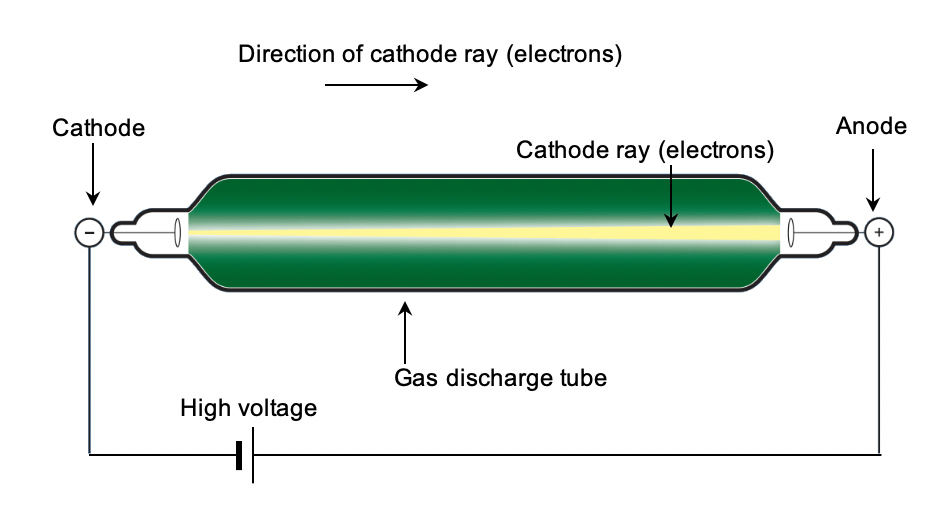### Doppler effect

The Doppler effect refers to an apparent change in frequency of a wave due to relative motion between source and observer.

As a result of the motion of stars, the observed frequency of the light changes due to a phenomenon called the Doppler effect.

The observed frequency of light $f'$ will differ from the emitted frequency $f$ depending on whether the star and Earth move towards or away from each other and their relative speed:

$\Large f'=f \frac{(v_{wave}+v_{observer} ) }{(v_{wave}-v_{source})}$

### Eddy currents

Eddy currents are circulating induced currents that are set up in bulk pieces of metal experiencing a change in flux.

### Einstein’s Special Theory of Relativity

Einstein’s Special Theory of Relativity states that:

1. The laws of physics are the same in all inertial frames of reference. [Principle of relativity.]
2. The speed of light in vacuum has the same value for all observers, regardless of their state of motion or the state of motion of the source.

In Einstein’s Special Theory of Relativity, it is the speed of light that is absolute (same for all observers) and space and time change to accommodate this.

• The length of an object measured depends on the relative motion of the observer. Hence length or space is considered a relative concept.
• The time interval of an event measured depends on the relative motion of the observer. Hence time is considered a relative concept.

### Electric field ($E$)

An electric field is a region of influence around electric charges in which other charges experience an electric force. The electric field mediates the electric force (just like gravitational fields mediate the force of gravity).

### Electric potential difference (V)

Electric potential difference or potential difference or voltage V is defined as the work done per unit charge in moving a charge between two points. It is measured in the SI unit of volts (V).

### Electromagnetic braking

Electromagnetic braking refers to a braking system that uses electromagnetic forces set up by eddy currents in a rotating metal plate or disc to slow or stop motion.

### Electromagnetic induction or EMF ($\varepsilon$)

Electromagnetic induction refers to the production of an induced EMF (Electro-Motive Force) by the use of magnetic means only. EMF can be thought of as a voltage or potential difference, which is induced in a conductor as a result of the relative motion of a magnet and a coil (even though there are no batteries in the circuit).

### Electromagnetic spectrum

The electromagnetic spectrum is a family of waves with the following common properties:

• Transverse in nature.
• Travel at the speed of light, c ($3 \times 10^8 ms^{-1}$ ).
• Do not require a medium to propagate.

The electromagnetic spectrum is divided into seven categories depending on frequency or wavelength.

4. Visible light

### Escape velocity ($v_{esc}$)

Escape velocity is the minimum velocity needed at a given distance from the planet to escape from the gravitational field of that planet.

Escape velocity is given by:

$\Large v_{esc}=\sqrt{\frac{2GM_{planet}}{r_{planet}}}$

### Faraday’s law of electromagnetic induction

Faraday’s law can be stated as follows:

An EMF is induced whenever a circuit experiences a change of magnetic flux and the EMF induced in a circuit is directly proportional to the rate of change of magnetic flux through the circuit.”

Mathematically,

$\Large \varepsilon=-\frac{N\Delta \Phi}{\Delta t}$

### Frequency ($f$)

Frequency is the number of revolutions completed by an object in one second. The unit of frequency is Hertz.

### Generators

Electric generators are mechanical devices that convert mechanical energy into electrical energy by the principle of electromagnetic induction.

There are two different types of generators:

1. AC generator produce alternating induced EMF and currents.
2. DC generators produce one-directional induced EMF and currents.

### Geostationary Earth Orbit (GEO)

A geostationary earth orbit is one in which the satellite is always in the same position with respect to the rotating Earth. In GEO, the velocity of the satellite relative to the ground is zero.

A hadron is a composite particle made of two or more quarks held together by the strong force.

### Hafele-Keating experiment

The Hafele-Keating experiment was conducted by Joseph Hafele and Richard Keating in 1971. It involved flying atomic clocks on aeroplanes to directly measure time dilation.

Three atomic clocks were used. All started in the same place at the United States Naval Observatory in Washington DC.

1. One atomic clock was left on the ground.
2. One atomic clock was flown around the world eastwards.
3. One atomic clock was flown around the world westwards.

The times on each clock were different as each clock experienced a different amount of time dilation.

• The eastward moving clock recorded a shorter time.
• The westwards moving clock recorded a longer time

The half-life is the time required for half of a radioactive sample to decay. Radioisotopes decay by emitting radiation. Therefore the quantity of a radioactive sample will decrease over time.

### Hyperfine spectral lines

When improved spectroscopic techniques were used to examine the spectral lines of hydrogen, it was found that many of the lines in the Balmer and other series were not single lines at all. Instead, each line was actually a pair of lines spaced very close together. This is known as hyperfine splitting.

Hydrogen spectral lines are shown below.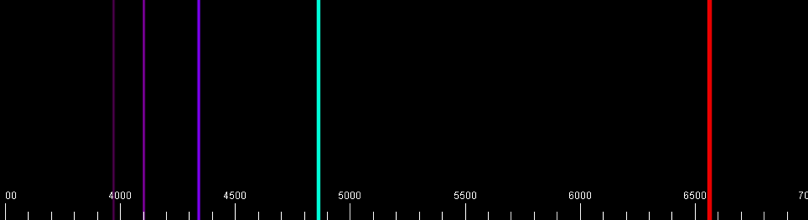By magnifying the above spectrum over a thousand times, we can see that the red spectral line is actually two spectral lines spaced very close together.### Inertia

Inertia is a measure of resistance to any changes in motion.

### Inertial frame of reference

An inertial frame of reference is a non-accelerating frame of reference in which an observer experience zero net force and moves with constant velocity or is at rest.

A train moving at a constant velocity is an example of an inertial frame of reference.

### Interference

Interference is a phenomenon in which two waves superpose to form a resultant wave of greater, lower, or the same amplitude. Depending on the relative phase of the superimposed waves we can constructive or destructive interference.

• If the waves add in phase they constructively interfere resulting in a larger amplitude wave (brighter region for light).
• If the waves add out of phase they destructively interfere resulting in smaller amplitude wave (darker region for light).

### Isotopes

The isotopes of an element have the same atomic number (proton number) but a different number of neutrons and hence a different mass number (protons + neutrons).

### Kepler’s Laws

Kepler proposed that the motion of the planets around the Sun can be described by the following three laws.

1. Kepler’s First Law: Each planet has an elliptical orbit with the Sun at one focus.
2. Kepler’s Second Law: Each planet moves so that a radius vector from the Sun to the planet sweeps out equal areas in equal times.
3. Kepler’s Second Law: The ratio of the cube of a planet’s average distance from the Sun to the square of a planet’s period of orbit has the same value for all the planets.Mathematically it can be expressed as: $\large \frac {r^3}{T^2} = k$

### Law of reflection

Light was known to reflect off a smooth surface (like a mirror) following the law of reflection, where the angle of incidence is equal to the angle of reflection.

The law of reflection is stated as: “The angle of reflection is equal to the angle of incidence.”

### Law of refraction (Snell’s Law)

Refraction is described by Snell’s Law, which relates the incidence angle $\theta_1$ ​ to the refracted angle $\theta_2$.​

Mathematically it is written as:

$\Large \frac{sin\theta_1}{sin\theta_2}=\frac{v_1}{v_2}$

### Lawrence cyclotron

The Lawrence cyclotron is a circular accelerator that produces accelerated charged particles in a circular path of increasing radius with the use of magnetic field.

### Length contraction

The length of an object measured in a reference frame in which the object is moving is always less than the proper length. The effect is known as length contraction

According to Einstein’s Special Theory of Relativity, the measured distance between two points depends on the frame of reference.

• The length of an object at rest with an observer is the proper length.
• The length of an object moving relative to an observer is the contracted length.

### Lenz’s law

Lenz’s law was first proposed by the German physicist Heinrich Lenz (1804 -1864) who formulated the direction in which EMF is induced in a conductor.

It is stated as follows : “The direction of the induced EMF in a circuit is such that it produces a current that will create its own magnetic field to oppose the change in magnetic flux responsible for producing it.

### Leptons

Leptons are a type of elementary particle that do not experience the strong force and hence cannot be held inside a nucleus.

### Linear velocity ($v$)

Linear velocity is the rate of change of linear displacement. It measures how fast or slow the linear displacement is changing with respect to time.

### Loop-de-loop

Loop-de-loop is a maneuver where a vehicle gains speed during a run up, and enters a vertical circular track that takes the vehicle upside down at the top of the circle, to upright again at the bottom of the circle.

### Low Earth Orbit (LEO)

A Low Earth Orbit (LEO) is an orbit in which a satellite orbits the Earth with altitudes of around 160 km – 1000 km. The International Space Station is in a LEO about 400 km above the Earth’s surface.

### Main Sequence star

Main Sequence stars are stars performing core hydrogen fusion and are in a state of of hydrostatic equilibrium where inward gravitational force is balanced by outward radiation pressure.

### Magnetic field ($B$)

A magnetic field is a model used to explain the region of influence within which a magnetic force may exist. Magnetic fields exist around magnets and around moving charges – like those found in current carrying conductors.

### Magnetic force on a moving charged particle ($F_{charge}$)

A charged particle moving in a magnetic field can experience a magnetic force. The magnitude of the magnetic force on a moving charged particle is given by:

$\large F=qv_{ \perp} B$

### Magnetic flux ($\Phi$)

The magnetic flux is visualised as a measure of the total number of magnetic field lines that pass through a chosen surface area.

The magnetic flux across a surface is determined  by the formula:

$\Large \Phi=B_{\parallel}A$

### Malus’ Law

Malus’s Law was discovered by Etienne-Louis Malus in 1809 and quantifies the extent to which a polariser allows a polarised beam of light to be transmitted.

The transmitted light intensity I is given by Malus’s Law:

$\Large I=I_{max}cos^2\theta$

where

• $I_{max}$ is the incident intensity
• $\theta$ is the angle between the incident polarisation and the polariser.

### Mass ($m$)

Mass is a measure of the inertia an object exhibits. It is measured in the SI unit of kilograms (kg).

### Mass dilation

An object in motion will have a greater mass than when it is stationary. This phenomenon is known as mass dilation. This means that mass is also a relative quantity.

Mass dilation is a consequence of relativistic momentum .

### Maxwells theory of electromagnetic waves

James C. Maxwell unified the phenomena of light and electromagnetism by developing the idea that light is a form of electromagnetic radiation .

Maxwell’s proposal regarding light consists of two parts:

1. Electromagnetic waves consist of a self-sustaining mutual generation of electric and magnetic fields that propagate at the speed of light.
2. Electric fields create magnetic fields, and magnetic fields create electric fields, resulting in a propagating wave. Visible light is an electromagnetic wave or electromagnetic oscillation

### Motor effect ($F_{conductor}$)

The phenomenon where a current carrying conductor in an external magnetic field experiences a force is known as the motor effect.

The  magnetic force on the current carrying conductor is given by

$\large F=LI_{\perp}B$

### Muons

Muons are unstable elementary particles, which have a charge equal to that of an electron and a mass 207 times greater.

### Non-inertial frame of reference

Non-inertial frame of reference is an accelerating frame of reference in which an observer experiences a net force and moves with increasing or decreasing velocity.

Examples of non-inertial frames of reference are:

• A train slowing down to a stop.
• An airplane taking off.

### Non-uniform circular motion

In a non-uniform circular motion, the speed of the object changes as it moves around a circular path in a fixed radius r. Objects rotating in a vertical plane is an example of non-uniform circular motion.

### Nuclear transmutation

Nuclear transmutation is a process of one element changing into another element through nuclear reactions.

### Orbital decay

Orbital decay refers to a process of losing energy due to atmospheric drag and undergoing a loss of altitude.

### Orbital velocity ($v_{orb}$)

Orbital velocity is the speed required to achieve orbit around a celestial body, such as a planet or a star.

The orbital speed of satellites around the Earth in a circular orbit is given by:

$\large v_{orb}=\sqrt{\frac{GM_{earth}}{r}}$

### Particle accelerators

Particle accelerators are devices used for accelerating elementary particles such as electrons and protons to high velocities and energies by means of electric or electromagnetic fields.

There are two basic types of particle accelerators:

1. Linear accelerators: Particles travel in a straight line path.
2. Circular accelerators: Particles travel in a circular path.

### Planck’s hypothesis

In 1901 Max Planck discovered a formula for blackbody radiation that was in complete agreement with experimental results at all wavelengths.

1. The energy of oscillating atoms that emit electromagnetic radiation is discrete or quantised. We say atoms will occupy discrete energy levels.
2. The frequency $f$ of radiation emitted is proportional to the amount of energy E released by an atom: $E=hf$

### Period ($T$)

Period is the time taken to complete one cycle/revolution. The SI unit of period is seconds.

### Photoelectric effect

The photoelectric effect (not photovoltaic effect!) is a phenomenon in which electrons are ejected from a metal when light is shone on the metal surface. Electrons emitted in the photoelectric effect are called photoelectrons.

### Photon theory of light

Einstein extended Planck’s concept of quantisation to electromagnetic waves and proposes the photon theory of light:

1. Light (or any electromagnetic wave) is a stream of particles known as photons.
2. Light energy is localised to each photon or discrete, not evenly spread across the wavefront of the light.
3. Each photon has an energy $E$, given by:

$\Large E= hf$

### Polarisation

Polarisation refers to the direction in which the electric field oscillates.

An ordinary beam of visible light is unpolarised, meaning the electric field will oscillate along different axes.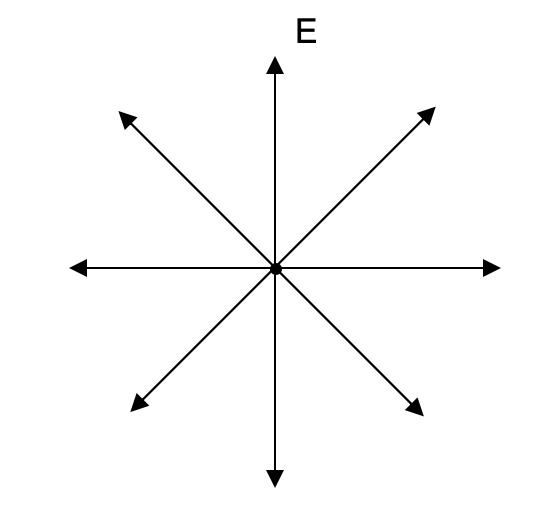When the oscillation of transverse waves is restricted to a single plane, the wave is linearly polarised.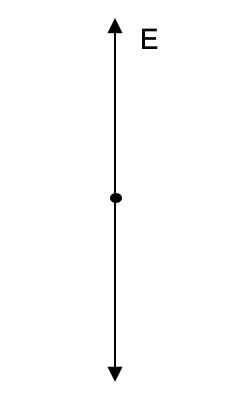### Principle of relativity

The principle of relativity was proposed by Galileo Galilei in 1632.

It states that: “ The mechanical laws of physics are the same for every observer moving uniformly with constant speed in a straight line (i.e. for every observer in an inertial frame).”

### Projectile

An object moving freely under the force of gravity is called a projectile.

### Projectile motion

Projectile motion is the two dimensional motion of an object thrown or projected into the air and moving only under the influence of gravitational force of attraction.

### Quarks

Quarks are a type of elementary particle which are usually found in combinations to form composite particles called hadrons such as protons and neutron.

### Red Giants

Red Giants are post main-sequence stars that have evolved from Main Sequence stars with masses less than 10 solar masses and are fusing helium into carbon in its core.

### Refraction of light

Refraction of light describes a wave bending as it moves from one medium to another. Refraction occurs because the speed of the wave changes when it enters the second medium. For the law of refraction, click here.

### Relativistic momentum

Relativistic momentum $p_v$ of an object is the momentum of an object moving at relativistic velocity. and depends on the reference frame of the observer.

Just like time and distance are relative quantities, momentum is also a relative quantity. An observer that is moving relative to an object will measure that object’s relativistic momentum.

$\Large p_v=\frac{m_0v}{\sqrt{1-\frac{v^2}{c^2}}}$

### Right Hand Palm Rule

Right Hand Palm Rule (RHPR) describes the relationship between the direction of the magnetic force on a positive charge, the velocity of the positive charge and the magnetic fields.

It can be used to determine the direction of the magnetic force on a moving positive charge. The Right Hand Palm Rule is stated below:

Open your right hand so that the fingers are together and the thumb sticks out. When your thumb is in the direction of v and, your fingers are in the direction of B then your palm faces in the direction of F.”### Rotational motion

Rotational motion is a rotation of an object about a point. Rotational motion can be circular or non-circular.

### Satellite

A satellite is an object that moves around a larger object, like the Earth or another planet.
There are two types of satellites that are in orbit around the Earth:

1. natural satellites like the Moon
2. artificial satellites like the satellites made by scientists and engineers to orbit the Earth for a variety of purposes.

### Schrödinger equation

In 1926 Austrian physicist Erwin Schrödinger published what is now known as the Schrödinger equation to describe wave mechanics, i.e. to describe how particles behave given their wave nature. ​

The Schrödinger equation for a hydrogen atom can be solved to find all the allowed states for the electron. These solutions are discrete and correspond to standing waves like in de Broglie’s case. However, these are now three-dimensional standing waves, which reflect the fact that electrons are free to move in three dimensions.

### Standard Model

Standard Model that explains our current understanding of the nature of matter:

• What are the components of matter?
• How do the components of matter interact with each other?

According to the Standard Model, all the particles in the universe can be grouped into just three “families” of elementary particles:

• 6 quarks, which combine to form nucleons and other particles
• 6 leptons, including the electron and neutrinos
• 5 force carrier particles, including the photon

### Strong nuclear force

Strong nuclear force is a fundamental force acting between nucleons and is responsible for the stability of nuclei. It is the nuclear binding force.

The strong nuclear force is responsible for the stability of nuclei. This force represents the “glue” that holds the nuclear constituents (nucleons) together and overcomes the electrostatic repulsion.

### Synchrotron

The synchrotron is a circular accelerator that produces accelerated particles at a constant orbital radius by synchronising the magnetic field strength with the energy of the accelerated particles.

Thermal radiation refers to light and heat emitted by an object due to its temperature.

### Time dilation

According to a stationary observer, a moving clock runs slower than an identical stationary clock. This effect is known as time dilation.

Time dilation is one of the consequences of Einstein’s Special Theory of Relativity – Time is a relative concept that depends on the motion of the observer.

### Torque ($\tau$)

Torque is defined as the tendency of a force to rotate an object about an axis. The magnitude of the torque is calculated by the following formula:

$\Large \tau=r_{\perp} F$

### Transformer

A transformer is an electrical device that uses electromagnetic induction to transfer energy from one AC system to another. A schematic diagram of an ideal single-phase transformer is shown below.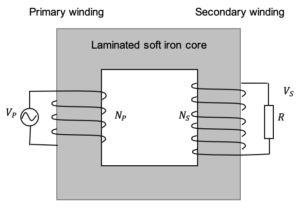### Transformer equation

In an ideal transformer, the ratio of primary to secondary voltages is equal to the ratio of primary to secondary turns.

$\Large \frac{V_p}{V_s}=\frac{N_p}{N_s}$

### Uniform circular motion

An object travelling in a circle at a constant speed is said to be undergoing circular motion.

### Van de Graaff accelerator

A Van de Graaff accelerator is a linear accelerator that produces accelerated particles in the form of positive ions (hydrogen ions) by stripping electrons from the hydrogen gas with a high positive voltage source.

### Weight

Weight  is the force with which an object is attracted to the Earth due to gravitational force. Weight and mass are related by the formula:

$\large W = mg$

### Weightlessness

An object is said to be weightless when its apparent weight is zero even though there is a force of gravity acting on it. Note that apparent weight is not the same as true weight mg.

### White Dwarfs

White Dwarfs are the remnant cores of stars that used to be Red Giants.

### Work function $\phi$

The work function, $\phi$ represents the minimum energy with which an electron is bound in the metal (usually a few electron volts).

The work function of a metal is related to the the threshold frequency:

$\Large \phi=hf_0$

### Zeeman effect

The Zeeman effect refers to the splitting up of spectral lines of atoms within the magnetic field. The simplest is the splitting up of one spectral line into three components called the “Normal Zeeman Effect”

## Want more detailed explanations than the glossary of Physics terms?